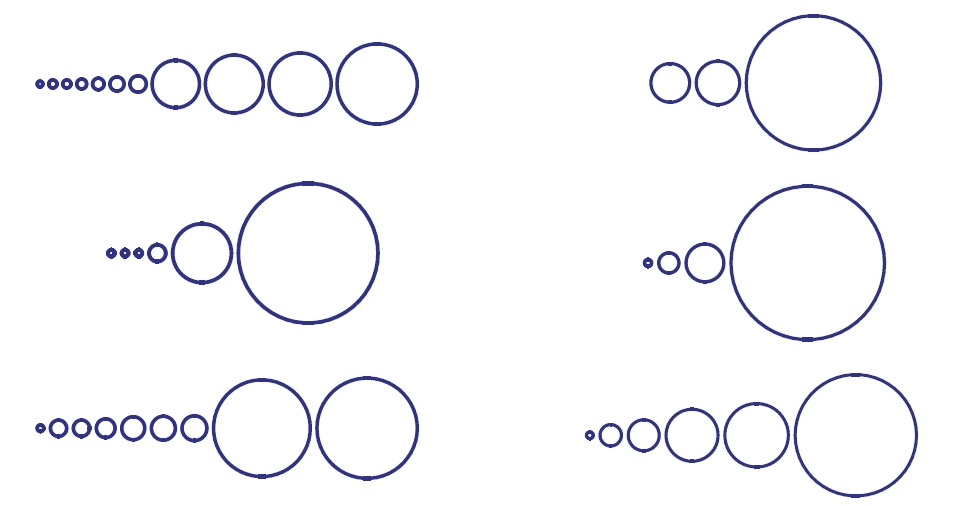Random Permutations (Part 7)

## Random Permutations (Part 7)

#### John Baez

Last time I computed the expected number of cycles of length $$k$$ in a random permutation of an $$n$$-element set. The answer is wonderfully simple: $$1/k$$ for all $$k = 1, \dots, n$$.

As Mark Meckes pointed out, this fact is just a shadow of a more wonderful fact. Let $$C_k$$ be the number of cycles of length $$k$$ in a random permutation of an $$n$$-element set, thought of as a random variable. Then as $$n \to \infty$$, the distribution of $$C_k$$ approaches a Poisson distribution with mean $$1/k$$.

But this is just a shadow of an even more wonderful fact!

First, if we fix some number $$b \ge 1$$, and look at all the random variables $$C_1, \dots, C_b$$, they converge to independent Poisson distributions as $$n \to \infty$$.

In fact we can even let $$b \to \infty$$ as $$n \to \infty$$, so long as $$n$$ outstrips $$b$$, in the following sense: $$b/n \to 0$$. The random variables $$C_1, \dots , C_b$$ will still converge to independent Poisson distributions.

These facts are made precise and proved here:

In the proof they drop another little bombshell. Suppose $$j \ne k$$. Then the random variables $$C_j$$ and $$C_k$$ become uncorrelated as soon as $$n$$ gets big enough for a permutation of an $$n$$-element set to have both a $$j$$-cycle and a $$k$$-cycle. That is, their expected values obey

$E(C_j C_k) = E(C_j) E(C_k)$

whenever $$n \ge j + k$$. Of course when $$n \lt j + k$$ it's impossible to have both a cycle of length $$j$$ and one of length $$k$$, so in that case we have

$E(C_j C_k) = 0$

It's exciting to me how the expected value $$E(C_j C_k)$$ pops suddenly from $$0$$ to $$E(C_j) E(C_k) = 1/jk$$ as soon as our set gets big enough to allow both a $$j$$-cycle and a $$k$$-cycle. It says that in some sense the presence of a large cycle in a random permutation does not discourage the existence of other large cycles... unless it absolutely forbids it!

This is a higher-level version of a phenomenon we've already seen: $$E(C_k)$$ jumps from $$0$$ to $$1/k$$ as soon as $$n \ge k$$.

More generally, Arratia and Tavaré show that

$E(C_{j_1} \, \cdots \, C_{j_m}) = E(C_{j_1}) \, \cdots \,E(C_{j_m})$

whenever $$n \ge j_1 + \cdots + j_m$$. But on the other hand, clearly

$E(C_{j_1} \cdots C_{j_m}) = 0$

whenever $$n \lt j_1 + \cdots + j_m$$, at least if the numbers $$j_i$$ are distinct. After all, it's impossible for a permutation of a finite set to have cycles of different lengths that sum to more than the size of that set.

I'm trying to understand the nature of random permutations. These results help a lot. But instead of describing how they're proved, I want to conclude with a picture that has also helped me a lot:Six random permutations of a 500-element set, where
circle areas are drawn in proportion to cycle lengths.
From Analytic Combinatorics by Flajolet and Sedgewick.

One can argue that Flajolet and Sedgewick should have used circumferences rather than areas to indicate cycle lengths: after all, the circumference is a kind of 'cycle length'. That would have made the large cycles seem even larger compared to the puny ones.

Of course, experts on the visual display of quantitative information have a lot of opinions on these issues. But never mind! Let's extract some morals from this chart.

We can see that a random permutation of a large set has rather few cycles on average. To be precise, the expected number of cycles in a random permutation of an $$n$$-element set is $$\sim \ln n$$.

We can also see that the lengths of the cycles range dramatically from tiny to large. To be precise, suppose $$1 \le m \le m' \le n$$. Then since the expected number of cycles of length $$k$$ is $$1/k$$, the expected number of cycles of length between $$m$$ and $$m'$$ is close to

$\ln m' \; - \; \ln m$

Thus we can expect as many cycles of length between $$1$$ and $$10$$ as between $$10$$ and $$100$$, or between $$100$$ and $$1000$$, etc.

So, I'm now imagining a random permutation of a large set as a way of chopping up a mountain into rocks with a wild range of sizes: some sand grains, some pebbles, some fist-sized rocks and some enormous boulders. Moreover, as long as two different sizes don't add up to more than the size of our mountain, the number of rocks of those two sizes are uncorrelated.

I want to keep improving this intuition. For example, if $$j \ne k$$ and $$n \ge j + k$$, are the random variables $$C_j$$ and $$C_k$$ independent, or just uncorrelated?